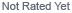Loading... Please wait...
• My Account

Currency Displayed in

Capacitor Values & Units

1 pF = .000001 μF

To convert pF to μF, multiply by .000001

Example: 4700 pF = .0047 μF

To convert μF to pF, multiply by 1,000,000

Example: .0033 μF = 3300 pF

Note: Many pieces of vintage equipment referred to capacitance units in micromicrofarads .

This unit is now referred to as picofarads (pF).

Capacitor Markings

The values of film and ceramic disk capacitors are typically marked in picofarads (pF) using a

3 digit number followed by a letter, for example:

682K

The first 2 digits are the two significant values of the capacitance and the 3rd digit is number of following zeroes.

For the above example, the value is 6800 pF = .0068 μF

The letter following the three digits is typically the value tolerance, where:

J = 5% K = 10% M = 20%

## New Products

•••••